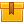# Introduction to Statistics (A4309)

## Prerequisites

This lesson is designed to build on the skills presented in the eighth lesson of this program, Introduction to Geometry.

## Description

This lesson provides an introduction to some basic principles of statistics. Emphasis is on statistical functions that are most commonly encountered in the workplace and everyday life. The topics covered include construction and interpretation of charts and graphs. The lesson also explains averages such as mean, median and mode and the appropriate use of each.

## Objectives

Recognize and interpret tally charts. Recognize and interpret bar graphs. Recognize and interpret pie charts. Recognize and interpret line graphs. Construct graphs and charts to display data. Explain the concept of central tendency. Calculate the mean for a sample. Find the mode of a sample. Explain the concept of variation. Calculate the range of a sample. Explain the concept of a normal curve Using a normal curve, explain the concept of standard deviation.

## ContentThis lesson provides an introduction to some basic principles of
statistics. Emphasis is on statistical functions that are most commonly
encountered in the workplace and everyday life.  The topics covered
include construction and interpretation of charts and graphs. The lesson
also explains averages such as mean, median and mode and the
appropriate use of each.

•Introduction to Statistics

0
0 Reviews